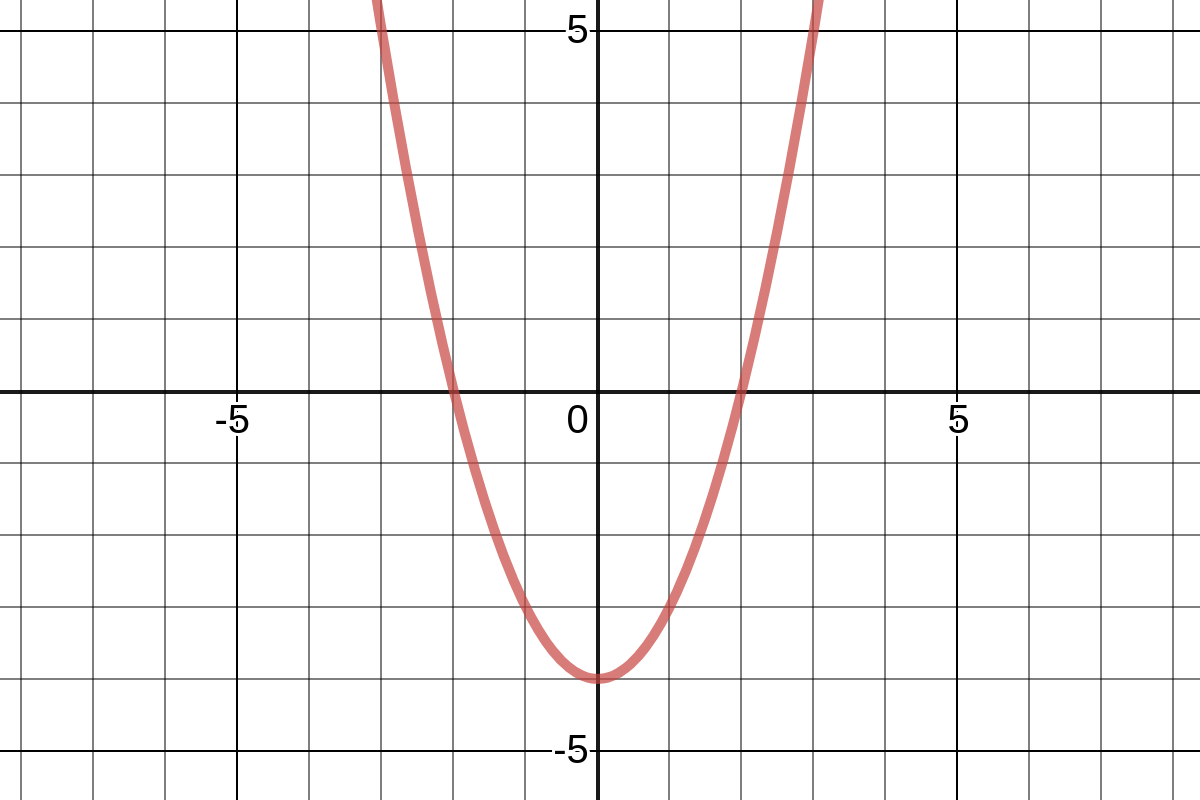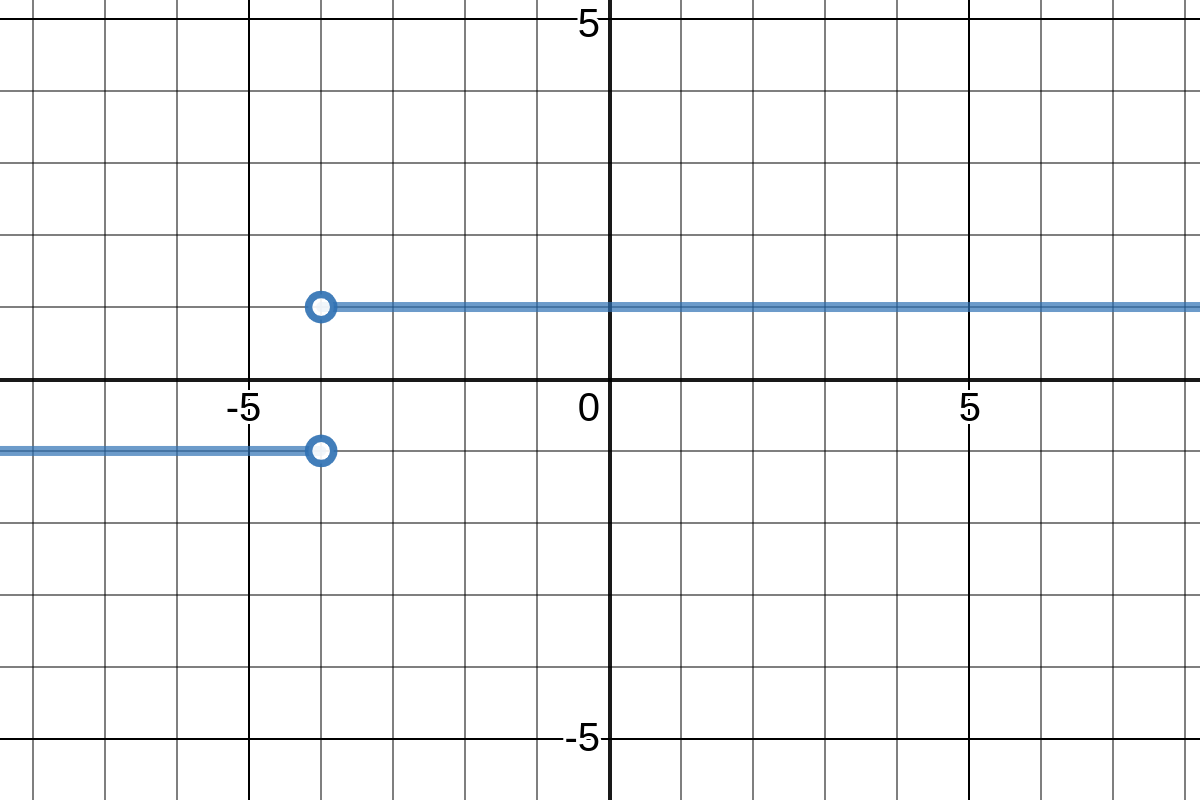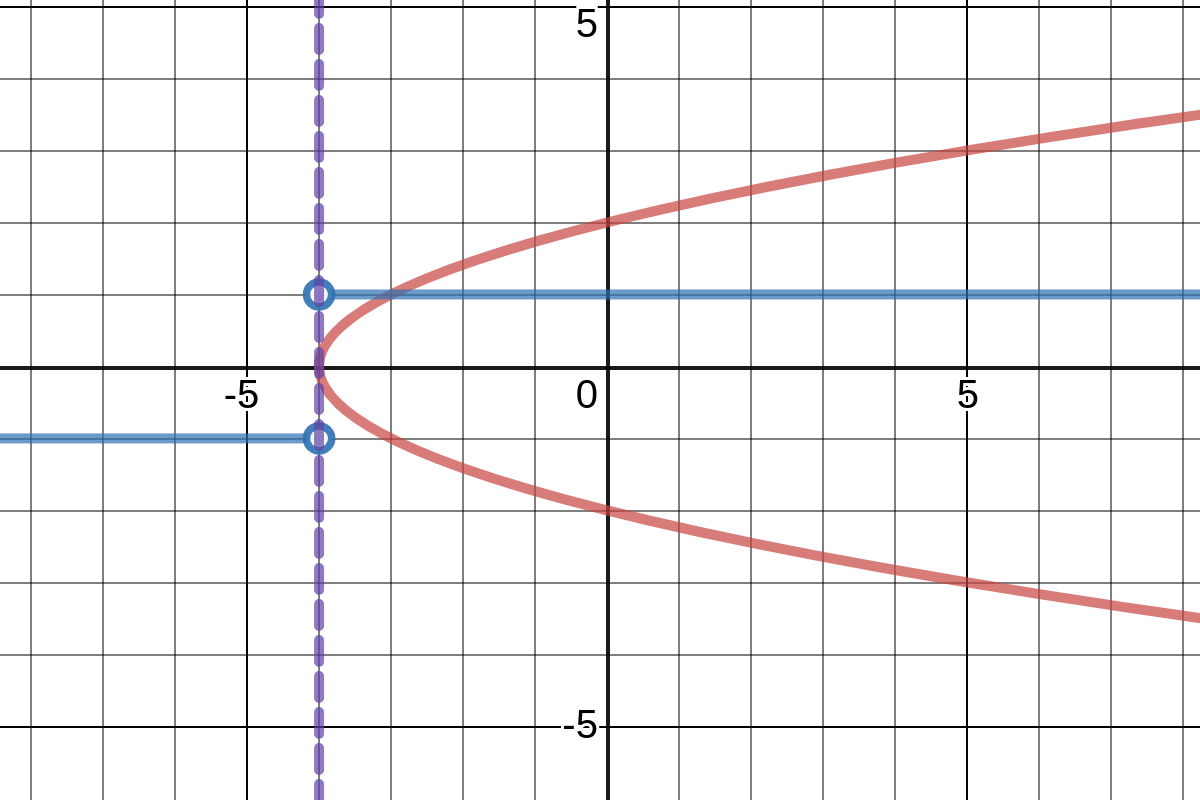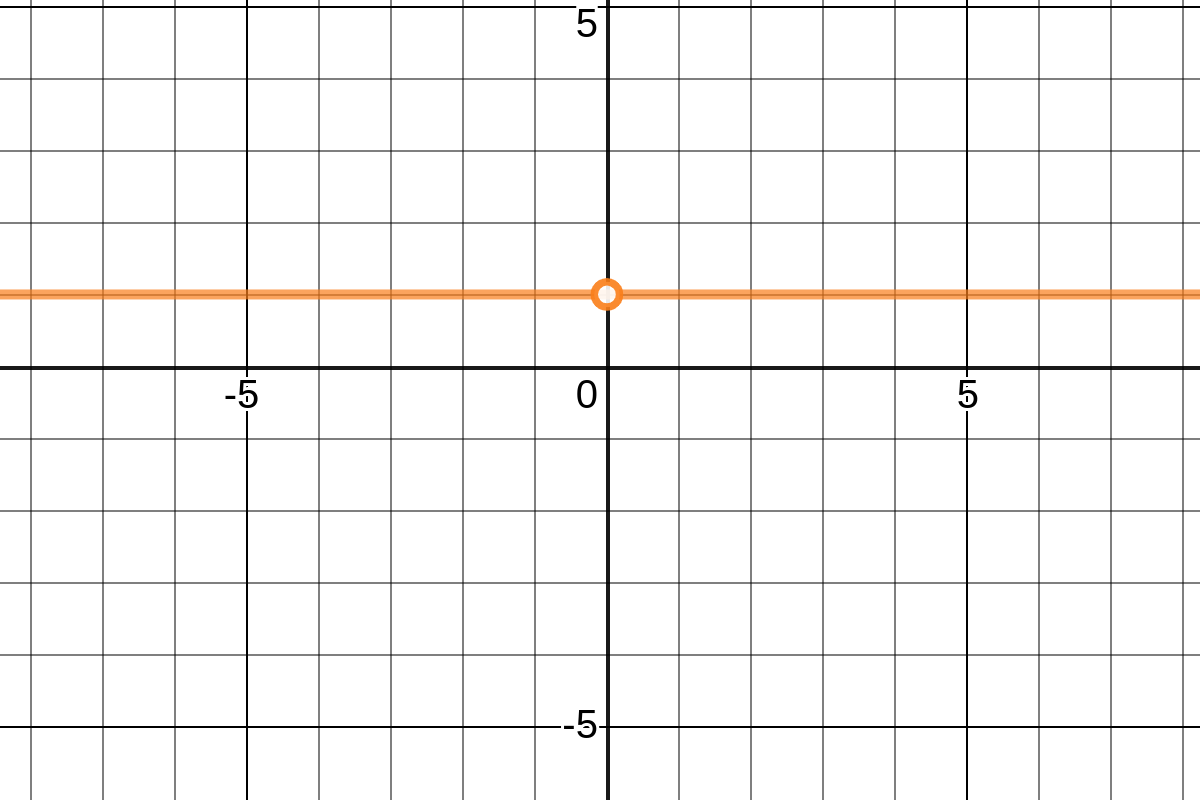# Resolving Limits Graphically Near Domain Of Discontinuity Via One-Sided Limits

A "fun" math article by Jack Giffin on 9/30/2019! Yay math!

🔙 to jackgiffin.com

### The Problem

You have a function $f\left(x\right)$ and a function $g\left(x\right)$ such as is shown below.

$f\left(x\right)$$g\left(x\right)$We readily know that $x\to 0f\left(x\right)=-4$, but how do we find $x\to 0g\left(f\left(x\right)\right)$? With direct substitution, we get $x\to 0g\left(f\left(x\right)\right)=x\to -4g\left(x\right)=DNE$ (DNE means Does Not Exist) because $x\to -4g\left(x\right)=-1$ and $x\to -4g\left(x\right)=1$, so $x\to -4g\left(x\right)\ne x\to -4g\left(x\right)$. Let us analyse what happens in function composition.

### The Solution Visually

Let us analyze the composition of $g\left(f\left(x\right)\right)$. Comprehend that $y=f\left(x\right)$ such that the domain—all x-values that the function passes through—is the input and the range—all y-values that the function passes through—is the output of the function. Now, imagine $g\left(f\left(x\right)\right)=g\left(y\right)$. From this perspective, the range outputted by $f\left(x\right)$ becomes the new domain of $g\left(f\left(x\right)\right)$. Observe the visual below. (Note that the grid is rotated 90 degrees to aid comprehension, so the x/y axes are flipped such that y is horizontal and x is vertical).

$y=f\left(x\right); g\left(f\left(x\right)\right)=g\left(y\right)$As the above image reveals, the range of $f\left(x\right)$ is never less than $-4$, so the numbers going into $g\left(y\right)$ are never less than $-4$. Thus, we can ignore all values of $g\left(y\right)$ that are less than $-4$, leaving $g\left(x\right)$ with only a single $y=1$ horizontal line which is the only remaining possible answer to $x\to 0g\left(f\left(x\right)\right)$, so $x\to 0g\left(f\left(x\right)\right)=1$ wins by default. Below is a graph of $g\left(f\left(x\right)\right)$. Notice how its range consists of a single number: 1. In other words, $g\left(f\left(x\right)\right)=1$ is true for each and every real value of x except for $0$ which is undefined.

$g\left(f\left(x\right)\right)$### The Solution Mathematically

Now, how to do $x\to 0g\left(f\left(x\right)\right)$ mathematically? Here's how:

1. Let $x\to cK\left(L\left(x\right)\right)$ represent the situation where $K\left(x\right)$ and $L\left(x\right)$ are both arbitrary functions that both pass the vertical line test.
2. Partial substitution with $K\left(x\to cL\left(x\right)\right)$ must have failed, else you would use this partial-substitution value as the result.
3. There must be a local minima or a local maxima at $L\left(c\right)$ such that either it's a local maxima due to $L\left(c\right)-1/\infty \le L\left(c\right) \le L\left(c\right)+1/\infty$ or it's a local minima due to $L\left(c\right)-1/\infty \ge L\left(c\right) \le L\left(c\right)+1/\infty$. In other words, the function must have either both higher or both lower values on either side of $c$. If there is no local minima/maxima, then $x\to cK\left(L\left(x\right)\right)=DNE$. Recall that infinitely small values can be used to prove one-sided limits such as $x\to 21+x/x-2=x\to 21+x/x\to 2x-2=2+1/x\to 2\left(x-1/\infty \right)-2=3/2-1/\infty -2=3/-1/\infty =-3\infty =-\infty$. These infinitely small values are also used with local minimas and local maximas in the same way. By an alternative definition, take the derivatives—rate of change at every single point—of $L\left(x\right)$ and there exists either a local minima or a local maxima at $c$ if $L\text{'}\left(c\right)=0$.
4. If there is a local minima—increasing values on either side—at $c$, then $x\to cK\left(L\left(x\right)\right)=y\to L\left(c\right)K\left(y\right)$. Else, if there is a local maxima—decreasing values on either side—at $c$, then $x\to cK\left(L\left(x\right)\right)=y\to L\left(c\right)K\left(y\right)$.